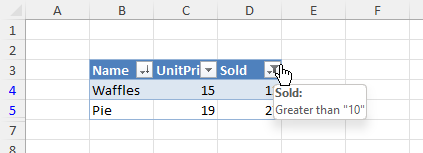# Tables

Excel can create a table from a range in a worksheet.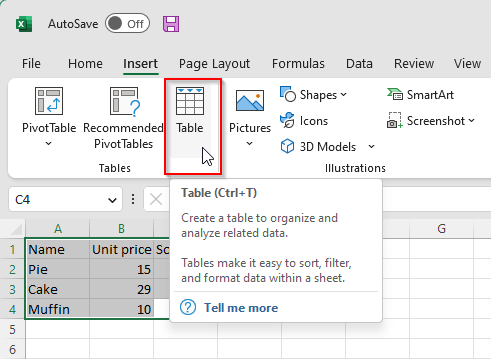## Table creation

Table can be created during data insertion or from existing data.

• IXLCell.InsertTable - create table when data is being inserted.

• IXLRange.CreateTable - create table from existing data.

Sample to create a tables
```using ClosedXML.Excel;

using var wb = new XLWorkbook();
ws.ColumnWidth = 12;
ws.FirstCell().InsertTable(new[]
{
new Pastry("Pie", 10),
new Pastry("Cake", 7),
new Pastry("Waffles", 17)
}, "PastrySales", true);

ws.Range("D2:D5").CreateTable("Table");

wb.SaveAs("tables-create.xlsx");

record Pastry(string Name, int Sales);
```

Since each column represents a field and each field must have a non-empty unique name in the table, the table creation methods will autogenerate a name for columns that are empty.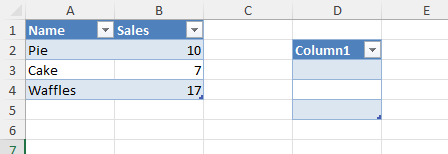## Table resizing

Table can be resized. If any header cell of a new range is empty, it will be filled with a auto-generated column name.

Sample to resize a table
```using ClosedXML.Excel;

using var wb = new XLWorkbook();

ws.Cell(1, 1).Value = "Foo";
ws.Cell(1, 2).Value = "Bar";
ws.Range("A2:B5").Value = 10;
var table = ws.Range("A1:B5").CreateTable();

table.Resize("B1", "D3");

wb.SaveAs("tables-resize.xlsx");
```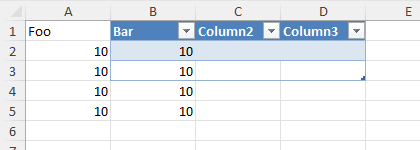## Table theme

Every table has a theme. A newly created table has a None theme. Table theme can be changed through IXLTable.Theme property to a member of XLThemeTable class. In order to map the XLThemeTable member to an Excel theme, use a tooltip that is displayed over the theme in the Excel.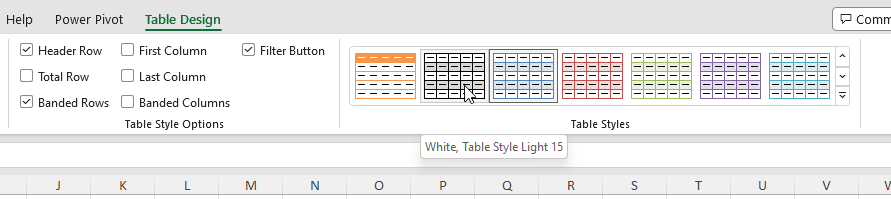Sample showing the table structure modification.
```using ClosedXML.Excel;

using var wb = new XLWorkbook();

ws.Cell("A1").SetValue("First");
ws.Cell("A2").InsertData(Enumerable.Range(1, 5));
ws.Cell("B1").SetValue("Second");
ws.Cell("B2").InsertData(Enumerable.Range(1, 5));

var table = ws.Range("A1:B6").CreateTable();
table.Theme = XLTableTheme.TableStyleLight16;

table = table.CopyTo(ws.Cell("D1")).CreateTable();
table.Theme = XLTableTheme.TableStyleDark2;

table = table.CopyTo(ws.Cell("G1")).CreateTable();
table.Theme = XLTableTheme.TableStyleMedium15;

wb.SaveAs("tables-themes.xlsx");
```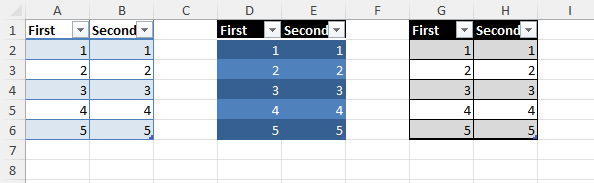## Table style options

Table has a style that can affect how it is displayed in a worksheet. IXLTable contains several properties that can modify how is the table displayed.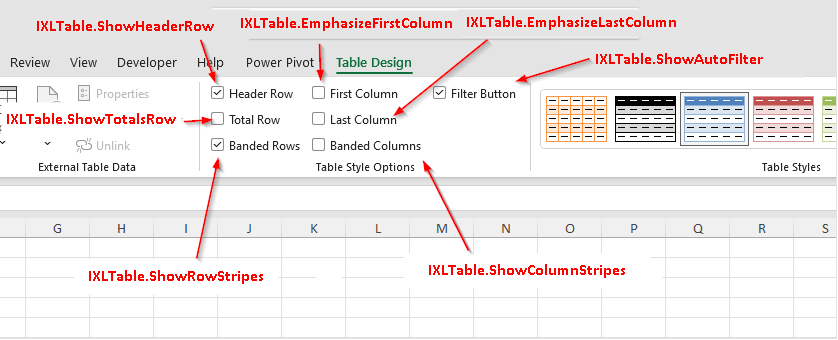Sample showing the table structure modification.
```using ClosedXML.Excel;

using var wb = new XLWorkbook();

ws.Cell("A1").SetValue("First");
ws.Cell("A2").InsertData(Enumerable.Range(1, 5));
ws.Cell("B1").SetValue("Second");
ws.Cell("B2").InsertData(Enumerable.Range(1, 5));

var table = ws.Range("A1:B6").CreateTable();
table.CopyTo(ws.Cell("D1")).CreateTable();

table
.SetShowRowStripes(false)
.SetShowColumnStripes(true)
.SetShowAutoFilter(true)
.SetShowTotalsRow(true);

// Totals can be set only if the totals row is displayed
table.Field("First").TotalsRowFunction = XLTotalsRowFunction.Sum;
table.Field("Second").TotalsRowFunction = XLTotalsRowFunction.Average;

wb.SaveAs("tables-structure.xlsx");
```

The sample sets the style options only for one table and has a second table one for comparison.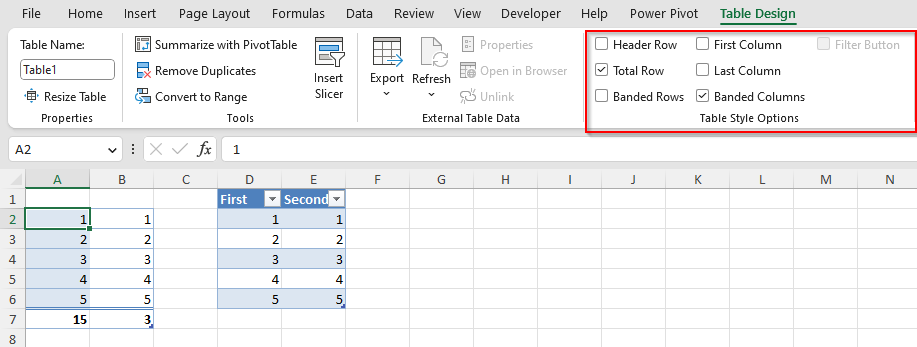## Table name

Table has a name. Name of the table must be unique across a worksheet. The table name can be used in formulas through structured references, e.g. =SUM(PastryTable[Sales]) instead of referencing column cells directly.

How to use table name in structural reference.
```using ClosedXML.Excel;

using var wb = new XLWorkbook();
ws.FirstCell().InsertTable(new[]
{
new Pastry("Pie", 10),
new Pastry("Cake", 7),
new Pastry("Waffles", 17)
}, "PastrySales", true);

ws.Cell("D2").Value = "Total pastry sales:";
ws.Cell("D3").FormulaA1 = "SUM(PastrySales[Sales])";

wb.SaveAs("tables-formula-reference.xlsx");

record Pastry(string Name, int Sales);
```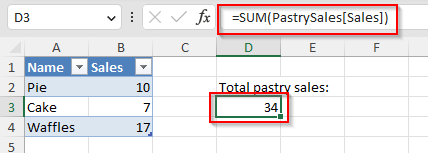You can change table name through IXLTable.Name property.

Warning

Evaluation of structured references is not yet supported.

Table can display a header row. Header row displays names of the table fields. Table field consists of data cells in a single column of the table. Whether the header row is displayed or not is determined by the IXLTable.ShowHeaderRow property.

ClosedXML table creation methods will use first values to deterine field names and will always display header by default (though it can be hidden).

Field names must be of type string. Field name can be changed either through IXLTableField.Name property or by setting a cell value, provided header column is shown. Cell value is converted to string though.

Individual table fields can be accessed through IXLTable.Field(string name) method or by IXLTable.Fields collection. There isn’t an indexer.

Header row is not the source of truth for field name, the field name is only projected to the header row cells. Fields have names, whether table has header row or not, as demonstrated by the sample.

```using ClosedXML.Excel;

using var wb = new XLWorkbook();

ws.Cell("A1").SetValue("Original Name");
ws.Cell("A2").InsertData(Enumerable.Range(1, 5));

var table = ws.Range("A1:A6").CreateTable("Table");
ws.Cell("A1").Value = "Changed Name";

// Reference original name of the field, although the actual value
// in the cell where header used to be is changed.
ws.Cell("D2").FormulaA1 = "SUM(Table[Original Name])";

```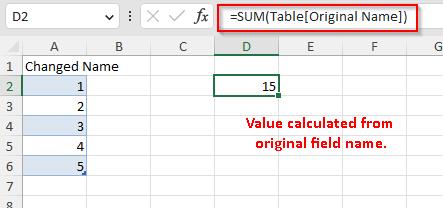## Totals

Table can display a totals row. Totals row usually contains formulas that summarize the field values. Whether the totals row is displayed or not is determined by the IXLTable.ShowTotalsRow property.

Methods that modify totals row will throw an exception if the table doesn’t show totals row.

```using ClosedXML.Excel;

using var wb = new XLWorkbook();

var table = ws.Cell("A1").InsertTable(new[]
{
new Pastry("Cake", 39, 3),
new Pastry("Waffles", 15, 12),
new Pastry("Pie", 19, 27)
});

table.ShowTotalsRow = true;
table.Field(nameof(Pastry.Name)).TotalsRowLabel = "Avg.";
table.Field(nameof(Pastry.UnitPrice)).TotalsRowFunction = XLTotalsRowFunction.Average;
table.Field(nameof(Pastry.Sold)).TotalsRowFunction = XLTotalsRowFunction.Average;

wb.SaveAs("tables-totals.xlsx");

record Pastry(string Name, double UnitPrice, int Sold);
```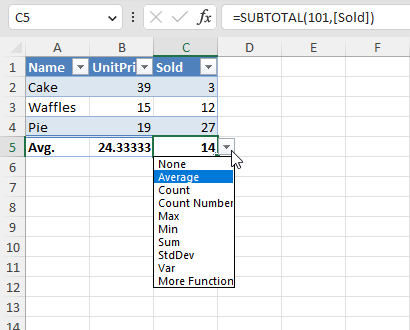It’s also possible to use a custom formula through IXLTableField.TotalsRowFormulaA1 property. Formula should reference table fields through structured references to ensure correctness even after table resizing.

## Auto Filter

Table can be filtered and sorted through auto filter feature. For more details, see autofilter documentation.

```using ClosedXML.Excel;

using var wb = new XLWorkbook();

var table = ws.Cell("B3").InsertTable(new[]
{
new Pastry("Cake", 39, 3),
new Pastry("Waffles", 15, 12),
new Pastry("Pie", 19, 27)
});

table.ShowAutoFilter = true;

// Must enable the filter
table.AutoFilter.IsEnabled = true;

// Sort by first column, desc
table.AutoFilter.Sort(1, XLSortOrder.Descending);

// Only pastries that sold more than 10 pieces (through column number in the table)
table.AutoFilter.Column(3).GreaterThan(10);

wb.SaveAs("tables-autofilter.xlsx");

record Pastry(string Name, double UnitPrice, int Sold);
```